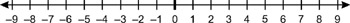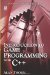# 3.1 1D Coordinates

## 3.1 1D Coordinates

The standard number line was demonstrated in the previous chapter. It can be thought of as a straight line with 0 in the center, positive numbers to the right, and negative numbers to the left. Using these numbers, along with fractions, any point along that line can be expressed as being some distance away from 0, such as 1, 3, or 7½. This is an example of a one-dimensional (1D) coordinate system, or graph. 0 is called the origin because it is the reference point, the starting location, from which any distance along the line is measured.Figure 3.1: 1D coordinatesIntroduction to Game Programming with C++ (Wordware Game Developers Library)
ISBN: 1598220322
EAN: 2147483647
Year: 2007
Pages: 225
Authors: Alan Thorn

Similar book on Amazon